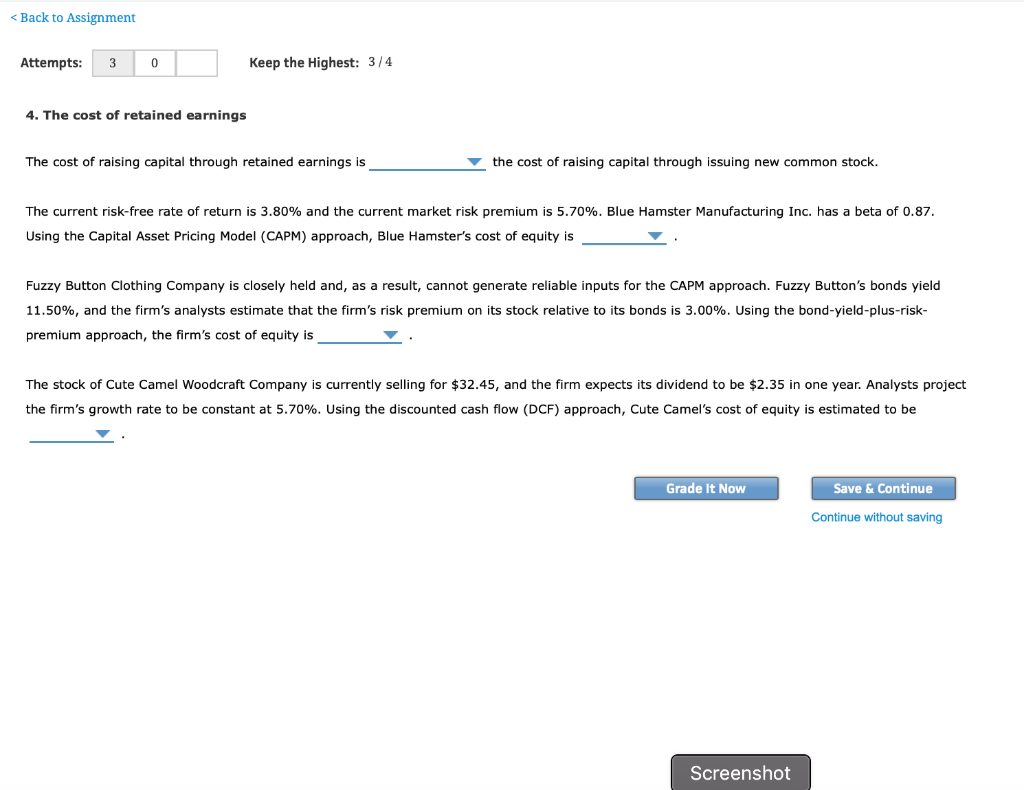# Back to Assignment Attempts: 30 Keep the Highest: 3/4 . The cost of retained earnings The cost of...Back to Assignment Attempts: 30 Keep the Highest: 3/4 . The cost of retained earnings The cost of raising capital through retained earnings is the cost of raising capital through issuing new common stock. The current risk-free rate of return is 3.80% and the current market risk premium is 5.70%. Blue Hamster Manufacturing Inc. has a beta of 0.87. Using the Capital Asset Pricing Model (CAPM) approach, Blue Hamster's cost of equity is Fuzzy Button Clothing Company is closely held and, as a result, cannot generate reliable inputs for the CAPM approach. Fuzzy Button's bonds yield 11.50%, and the firm's analysts estimate that the firm's risk premium on its stock relative to its bonds is 3.00%. Using the bond-yield-plus-risk- premium approach, the firm's cost of equity is The stock of Cute Camel Woodcraft Company is currently selling for \$32.45, and the firm expects its dividend to be \$2.35 in one year. Analysts project the firm's growth rate to be constant at 5.70%. Using the discounted cash flow (DCF) approach, Cute Camel's cost of equity is estimated to be Grade It Now Save & Continue Continue without saving Screenshot

a) The cost of raising capital through retained earning is less costlier /less expensive than cost of raising through issuing new equity

B) cost of equity =risk free rate+(beta*market risk premium)

= 3.8 + (.87*5.7)

=3.8+ 4.96

= 8.76%

C) cost of equity =Bond yield +risk premium

= 11.5 +3

= 14.5%

D) cost of equity=(D1/price)+growth

(2.35/32.45) +.057

.0724 +.057

.1294 or 12.94%

##### Add Answer of: Back to Assignment Attempts: 30 Keep the Highest: 3/4 . The cost of retained earnings The cost of...
More Homework Help Questions Additional questions in this topic.

• #### Cost of equity from retained earnings

Need Online Homework Help?